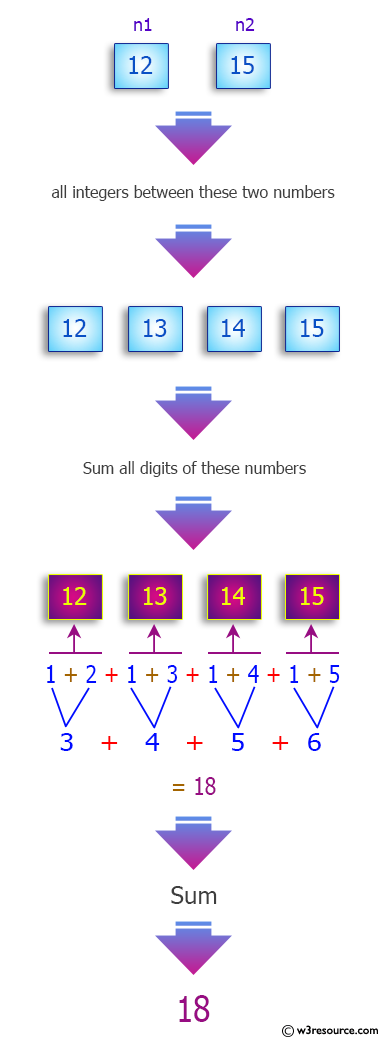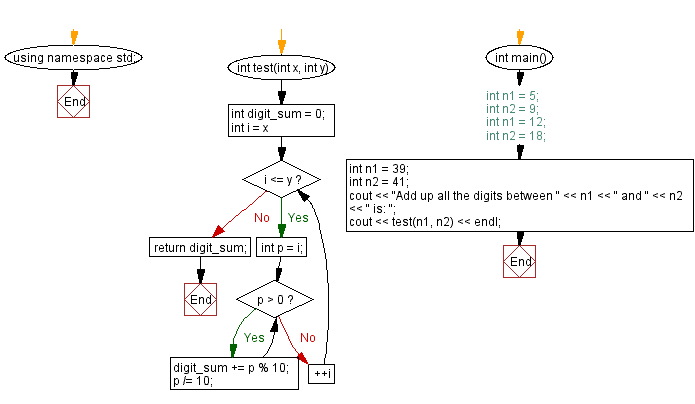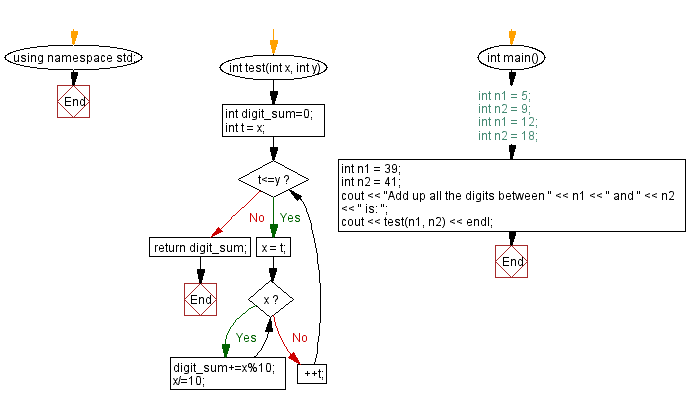﻿ C++ - Add up all the digits between two numbers

# C++ Exercises: Add up all the digits between two numbers

## C++ Basic: Exercise-86 with Solution

Write a C++ program to add up all the digits between two given integers.
Add all the digits between 11 and 16.
// 11, 12, 13, 14, 15, 16
(1 + 1) + (1 +2) + (1 + 3) + (1 + 4) + (1 + 5) + (1 + 6)
= 2 + 3 + 4 + 5 + 6 + 7
= 27

Sample Data:

(5, 9) -> 35
(12, 18) -> 42
(39, 41) -> 21

Pictorial Presentation:Sample Solution-1:

C++ Code :

``````#include <iostream>

using namespace std;
int test(int x, int y)
{
int digit_sum = 0;
for(int i = x; i <= y; ++i)
{
int p = i;
while(p > 0)
{
digit_sum += p % 10;
p /= 10;
}
}
return digit_sum;
}

int main() {
//int n1 = 5;
//int n2 = 9;
//int n1 = 12;
//int n2 = 18;
int n1 = 39;
int n2 = 41;
cout << "Add up all the digits between " << n1 << " and " << n2 << " is: ";
cout << test(n1, n2) << endl;
}
``````

Sample Output:

```Add up all the digits between 39 and 41 is: 21
```

Flowchart:Sample Solution-2:

C++ Code :

``````#include <iostream>

using namespace std;
int test(int x, int y)
{
int digit_sum=0;
int t = x;
while(t<=y){
x = t;
while(x){
digit_sum+=x%10;
x/=10;
}
++t;;
}
return digit_sum;
}

int main() {
//int n1 = 5;
//int n2 = 9;
//int n1 = 12;
//int n2 = 18;
int n1 = 39;
int n2 = 41;
cout << "Add up all the digits between " << n1 << " and " << n2 << " is: ";
cout << test(n1, n2) << endl;
}
``````

Sample Output:

```Add up all the digits between 39 and 41 is: 21
```

Flowchart:C++ Code Editor:

Previous C++ Exercise: Find the total number of minutes between two times.
Next C++ Exercises: C++ For Loop Exercises Home.

What is the difficulty level of this exercise?

﻿

## C++ Programming: Tips of the Day

Why is there no std::stou?

The most pat answer would be that the C library has no corresponding "strtou", and the C++11 string functions are all just thinly veiled wrappers around the C library functions: The std::sto* functions mirror strto*, and the std::to_string functions use sprintf.

Ref: https://bit.ly/3wtz2qA

We are closing our Disqus commenting system for some maintenanace issues. You may write to us at reach[at]yahoo[dot]com or visit us at Facebook